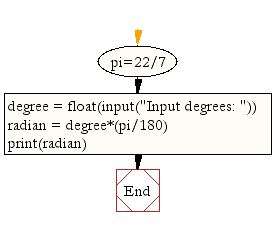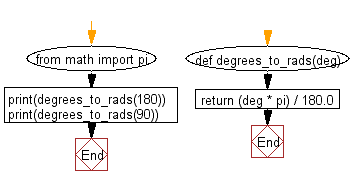﻿ Python Math: Convert degree to radian - w3resource# Python Math: Convert degree to radian

## Python Math: Exercise-1 with Solution

Write a Python program to convert degree to radian.
Note : The radian is the standard unit of angular measure, used in many areas of mathematics. An angle's measurement in radians is numerically equal to the length of a corresponding arc of a unit circle; one radian is just under 57.3 degrees (when the arc length is equal to the radius).

Test Data:
Degree : 15

Sample Solution-1:

Python Code:

``````pi=22/7
degree = float(input("Input degrees: "))
```
```

Sample Output:

```Input degrees: 90
1.5714285714285714
```

Pictorial Presentation:Flowchart:## Visualize Python code execution:

The following tool visualize what the computer is doing step-by-step as it executes the said program:

Sample Solution-2:

Use math.pi and the degrees to radians formula to convert the angle from degrees to radians.

Python Code:

``````from math import pi
return (deg * pi) / 180.0
```
```

Sample Output:

```3.141592653589793
1.5707963267948966
```

Flowchart:## Visualize Python code execution:

The following tool visualize what the computer is doing step-by-step as it executes the said program:

Python Code Editor:

Have another way to solve this solution? Contribute your code (and comments) through Disqus.

What is the difficulty level of this exercise?

Test your Programming skills with w3resource's quiz.

﻿

```>>> students = [{'name': 'John', 'score': 98}, {'name': 'Mike', 'score': 94}, {'name': 'Jennifer', 'score': 99}]